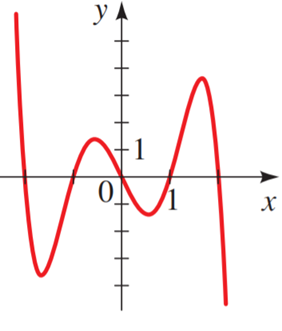# To match : the polynomial function### Precalculus: Mathematics for Calcu...

6th Edition
Stewart + 5 others
Publisher: Cengage Learning
ISBN: 9780840068071### Precalculus: Mathematics for Calcu...

6th Edition
Stewart + 5 others
Publisher: Cengage Learning
ISBN: 9780840068071

#### Solutions

Chapter 3.2, Problem 11E
To determine

## To match: the polynomial function

Expert Solution### Explanation of Solution

Given information:

The polynomial function is R(x)=x2+5x34

The power of the equation is odd and the leading co-efficient negativeso, predict the end behaviour, which is when x approaches positive , y will approach negative and as x approaches negative , y will approach positive .GraphVand IV contain this characteristic. Now substitute x with any value

12+5(1)34                          [substitute the value of x]1+54                                      [evaluate power]55                                     0                                                    [subtract]

Therefore, the coordinate point (1, 0 ),the graph Vcontain this closest

Hence, the correct answer is V.

### Have a homework question?

Subscribe to bartleby learn! Ask subject matter experts 30 homework questions each month. Plus, you’ll have access to millions of step-by-step textbook answers!